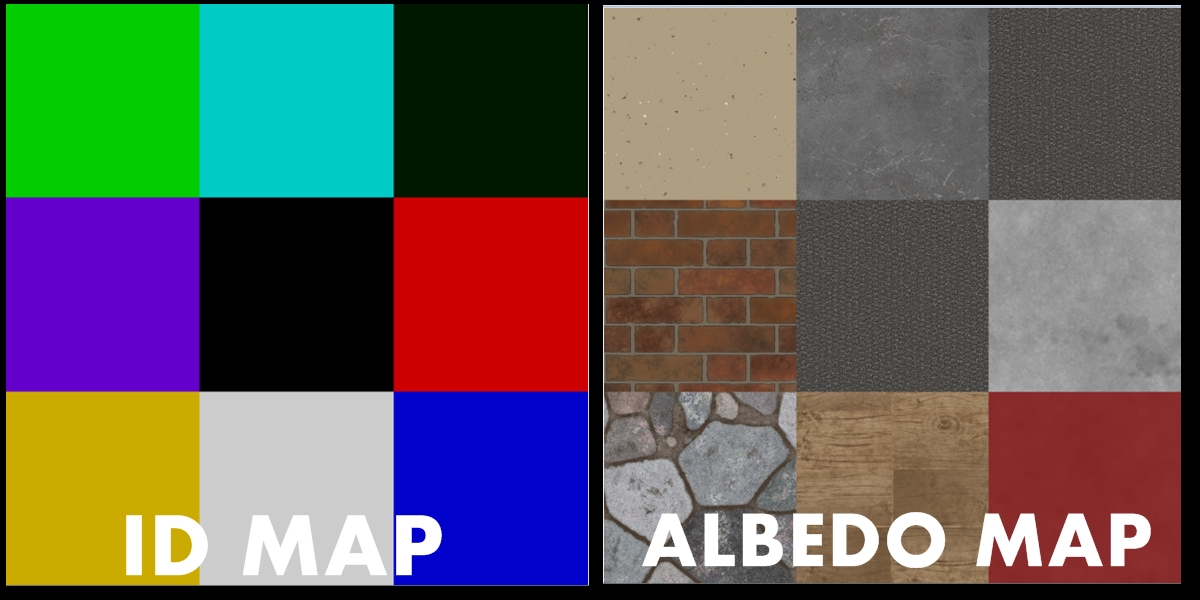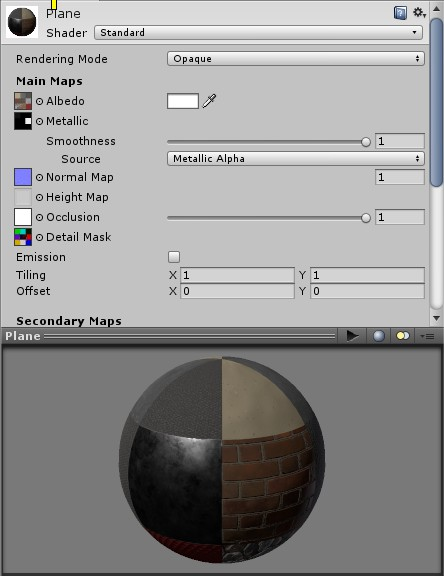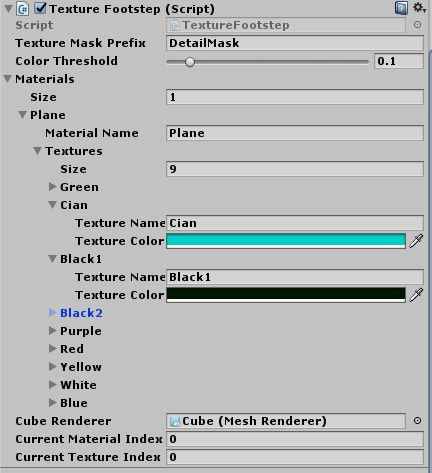Unity Tutorial / Explanation: Using masks and colors to improve the detection of object surfaces

There are several tutorials in Unity for how to recognize the surface of an object using a trigger collider or using raycasts, some techniques will even get the index of terrain texture for footsteps, but when you want to get the reference of 1 type of texture between several in 1 material (or more) assigned to an object? In this article I will show you how to use the properties and color classes and masks of materials of unity applied to the mesh for example making a footstep in a plane.

Posted by on - Intermediate Other

In this article I will show in the Unity tool how to improve the detection of the materials or textures by the collisions using a mask and a color reference. In my game (Brave Battle) I was frustrated at having to for every surface to have by a collider to detect its property in order to play a sound of footsteps or use exact particles. Taking a closer look at the internet, I found the FMOD (sound effects engine) tutorial where it was shown how to make a footsteps system using a mask.

With this I improved on this idea, expanding it so that I can recognize where each texture is in 1 single material using as base a mask (DetailMask) filled with the color id of the texture of the object and doing a color reference with a small database.

In the image below is shown the texture id map and albedo color, this process is to have a single material with multiple textures (Substance Painter and Blender used in this textures):Here below the id map image assigned to the Input Detail Mask of its respective material:How the process works:

In unity there is a function / method in the texture class that returns a filtered pixel color at normalized coordinates (UV). Using this to get the color of the mask (DetailMask) and make a reference comparing the RGB values of the returned color to a color saved in a database / color list, so if the returned color is equal to the reference color, it can be for example "wood equals to red color", can execute the surface event (footstep, effect, particle ...).

First we throw a raycast on the floor to get the material, we check the index of the material by GetTriangles method and once we have the correct material, we call the GetPixelBilinear method:

``````RaycastHit hit;
if (Physics.Raycast(transform.position, -Vector3.up, out hit, Mathf.Infinity)) { //Cast a raycast from player to ground
int materialIndex = GetMaterialIndex(hit); //check current material hitted (if more then one)
if (materialIndex != -1) { //Check if returned index value is not null
Material material = hit.collider.transform.GetComponent<Renderer>().materials[materialIndex]; //references the index with the getted material
if(material.name == "Plane (Instance)"){ //Instance is because on runtime unity creates a new object for material
hittedColor = GetPixelBilinear(material, hit.textureCoord, textureMaskPrefix); //Saves returned color in a variable
}
}
}``````
``````public static int GetMaterialIndex (RaycastHit hit) {
Mesh m = hit.collider.gameObject.GetComponent<MeshFilter>().mesh;
int[] triangle = new int[]
{
m.triangles[hit.triangleIndex * 3 + 0],
m.triangles[hit.triangleIndex * 3 + 1],
m.triangles[hit.triangleIndex * 3 + 2]
};
for(int i = 0; i < m.subMeshCount; ++i)
{
int[] triangles = m.GetTriangles(i);
for(int j = 0; j < triangles.Length; j += 3)
{
if(triangles[j + 0] == triangle &&
triangles[j + 1] == triangle &&
triangles[j + 2] == triangle)
return i;
}
}
return -1;
}``````
``````public static Color GetPixelBilinear (Material material, Vector2 position, string textureName) { //Pass parameters
Texture2D maskTexture = material.GetTexture("_" + textureName) as Texture2D; //Saves in a variable the correct texture mask
return maskTexture.GetPixelBilinear(position.x, position.y); //Return a pixel color bilinearly filtered
}``````

In the code, we get 1 pixel color (RGB) of a mask of the material in Color format. For more info: Docs.unity3d.com

``````if (myReferColor.Equals(hittedColor)) {
Debug.Log("Color: " + hittedColor.ToString());
}``````

We could use Equals to compare the colors but it would return the exact value of the RGB values, and sometimes because it is accurate it does not recognize the color. The solution is to create a method to get the approximate value of the RGB values using a threshold for margin of error:

``````public static bool ColorApproximately(Color a, Color b, float threshold) {
return ((a.r - b.r) < 0 ? ((a.r - b.r) * -1) : (a.r - b.r)) <= threshold
&& ((a.g - b.g) < 0 ? ((a.g - b.g) * -1) : (a.g - b.g)) <= threshold
&& ((a.b - b.b) < 0 ? ((a.b - b.b) * -1) : (a.b - b.b)) <= threshold;
}``````

That way we can make the reference of the color taken by raycast to a variable that we already have, and by comparing them we can carry out an event. A good implementation is to create a list that references colors and its name or sound for example to have all the references of the texture of the object. Here's an implementation made like this:Below a video showing the functional concept could easily be implemented for the hit effect of a gun or footsteps:

For doubts send an email to me, it is not every time I can respond because of the area that I live that sometimes does not have access to the internet, but if you see the email I respond. Email: thomaspontes13@gmail.com